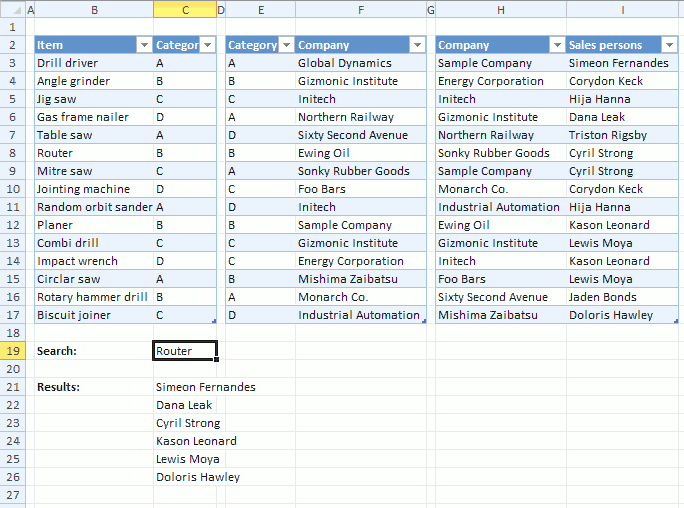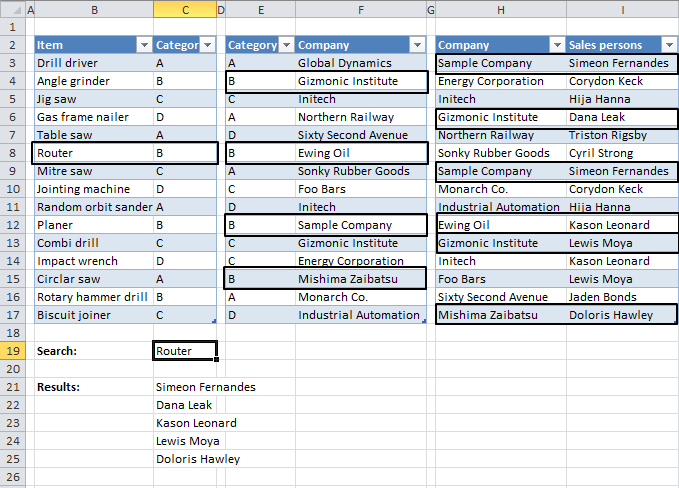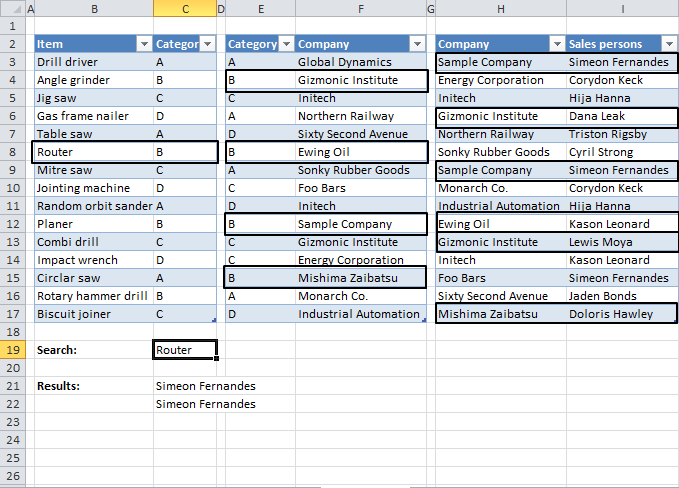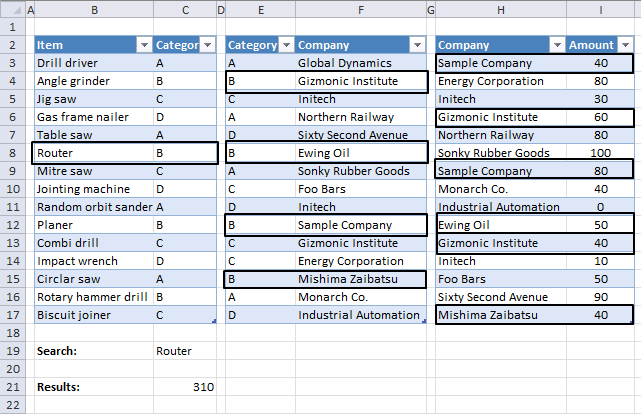Author: Oscar Cronquist Article last updated on October 17, 2019I will in this article demonstrate four formulas that do lookups, extract unique distinct and duplicate values and sums numbers across three relational data sets using Excel formulas.

The image above shows a search value in cell C19, it is used as a search condition in column B and a matching value is found in cell B8. The corresponding value in column C is cell C8 which is then used do a second lookup in another table next to the first table.

The value in cell C8 is found in column E four times, they are E4, E8, E12 and E15. The formula now uses the values on the same row in column F to do a third lookup in column H.

Six matches are found in column H and the values on the same rows from colmn I are returned to cell range C21:C26.

#### What is a relational table?

In a relational database (Microsoft Access), the data in one table is related to the data in other tables. In general, tables can be related in one of three different ways: one-to-one, one-to-many or many-to-many.  The relationship is used to cross-reference information between tables.
Source: University of Sussex

### Lookups in three related tables and return multiple valuesThe animated picture above shows you how the array formula works, it looks for the Item "Router" in Item column in the first table (column B and C). The corresponding Category is B.

In table two (column E and F) there are 4 matches to Category "B". The corresponding companies in table 2 are "Gizmonic Institute", "Ewing Oil", "Sample Company" and "Mishima Zaibatsu".

In table 3 (column H and I) there are six matches to category "Company" and the adjacent Sales persons are returned in cell range C21:C27.

Array formula in cell range C21:

=IFERROR(INDEX(Table3[Sales persons], SMALL(IF(ISERROR(MATCH(Table3[Company], IF(ISERROR(MATCH(Table2[Category], IF(\$C\$19=Table1[Item], Table1[Category], ""), 0)), "", Table2[Company]), 0)), "", MATCH(ROW(Table3[Sales persons]), ROW(Table3[Sales persons]))), ROW(A1))), "")

How to create an array formula

1. Copy above array formula
2. Select cell C21
3. Press with left mouse button on in formula bar4. Paste array formula (Ctrl + v)
5. Press and hold Ctrl+ Shift
6. Press Enter
7. Release all keys

The array formula is now surrounded by curly brackets, like this: {=array_formula}

How to copy array formula

1.  Select cell C21
2. Copy cell (Ctrl + c)
3. Select cell range C22:C27
4. Paste (Ctrl + v)

### Explaining lookup array formula in cell C21

#### Step 1 - Check whether a condition is met and return corresponding Table1[Category] value

IF(\$C\$19=Table1[Item],Table1[Category],"")

becomes

IF("Router" = {"Drill driver"; "Angle grinder"; "Jig saw"; "Gas frame nailer"; "Table saw"; "Router"; "Mitre saw"; "Jointing machine"; "Random orbit sander"; "Planer"; "Combi drill"; "Impact wrench"; "Circlar saw"; "Rotary hammer drill"; "Biscuit joiner"}, {"A"; "B"; "C"; "D"; "A"; "B"; "C"; "D"; "A"; "B"; "C"; "D"; "A"; "B"; "C"} , "")

and returns

{"";"";"";"";"";"B";"";"";"";"";"";"";"";"";""}

#### Step 2 - Return the relative position of an item in an array that matches a specified value

MATCH(Table2[Category], IF(\$C\$19=Table1[Item], Table1[Category], ""), 0)

becomes

MATCH(Table2[Category], {"";"";"";"";"";"B";"";"";"";"";"";"";"";"";""}, 0)

becomes

MATCH({"A"; "B"; "C"; "A"; "D"; "B"; "A"; "C"; "D"; "B"; "C"; "C"; "B"; "A"; "D"}, {"";"";"";"";"";"B";"";"";"";"";"";"";"";"";""}, 0)

and returns

{#N/A; 6; #N/A; #N/A; #N/A; 6; #N/A; #N/A; #N/A; 6; #N/A; #N/A; 6; #N/A; #N/A}

#### Step 3 -  Check whether a condition is met and return corresponding Table2[Company] value

IF(ISERROR(MATCH(Table2[Category], IF(\$C\$19=Table1[Item], Table1[Category], ""), 0)), "", Table2[Company])

becomes

IF(ISERROR({#N/A; 6; #N/A; #N/A; #N/A; 6; #N/A; #N/A; #N/A; 6; #N/A; #N/A; 6; #N/A; #N/A}), "", Table2[Company])

becomes

IF(ISERROR({#N/A; 6; #N/A; #N/A; #N/A; 6; #N/A; #N/A; #N/A; 6; #N/A; #N/A; 6; #N/A; #N/A}), "", {"Global Dynamics"; "Gizmonic Institute"; "Initech"; "Northern Railway"; "Sixty Second Avenue"; "Ewing Oil"; "Sonky Rubber Goods"; "Foo Bars"; "Initech"; "Sample Company"; "Gizmonic Institute"; "Energy Corporation"; "Mishima Zaibatsu"; "Monarch Co."; "Industrial Automation"})

and returns

{""; "Gizmonic Institute"; ""; ""; ""; "Ewing Oil"; ""; ""; ""; "Sample Company"; ""; ""; "Mishima Zaibatsu"; ""; ""}

#### Step 4 - Return the relative position of an item in an array that matches a specified value

MATCH(Table3[Company], IF(ISERROR(MATCH(Table2[Category], IF(\$C\$19=Table1[Item], Table1[Category], ""), 0)), "", Table2[Company]), 0)

becomes

MATCH({"Sample Company"; "Energy Corporation"; "Initech"; "Gizmonic Institute"; "Northern Railway"; "Sonky Rubber Goods"; "Sample Company"; "Monarch Co."; "Industrial Automation"; "Ewing Oil"; "Gizmonic Institute"; "Initech"; "Foo Bars"; "Sixty Second Avenue"; "Mishima Zaibatsu"}, {""; "Gizmonic Institute"; ""; ""; ""; "Ewing Oil"; ""; ""; ""; "Sample Company"; ""; ""; "Mishima Zaibatsu"; ""; ""}, 0)

and returns

{10; #N/A; #N/A; 2; #N/A; #N/A; 10; #N/A; #N/A; 6; 2; #N/A; #N/A; #N/A; 13}

#### Step 5 - Check whether a condition is met and return row number

IF(ISERROR(MATCH(Table3[Company], IF(ISERROR(MATCH(Table2[Category], IF(\$C\$19=Table1[Item], Table1[Category], ""), 0)), "", Table2[Company]), 0)), "", MATCH(ROW(Table3[Sales persons]), ROW(Table3[Sales persons])))

becomes

IF(ISERROR({10; #N/A; #N/A; 2; #N/A; #N/A; 10; #N/A; #N/A; 6; 2; #N/A; #N/A; #N/A; 13}), "", MATCH(ROW(Table3[Sales persons]), ROW(Table3[Sales persons])))

becomes

IF(ISERROR({10; #N/A; #N/A; 2; #N/A; #N/A; 10; #N/A; #N/A; 6; 2; #N/A; #N/A; #N/A; 13}), "", {1; 2; 3; 4; 5; 6; 7; 8; 9; 10; 11; 12; 13; 14; 15})

and returns

{1;"";"";4;"";"";7;"";"";10;11;"";"";"";15}

#### Step 6 - Return the k-th smallest row number

SMALL(array, k)

SMALL(IF(ISERROR(MATCH(Table3[Company], IF(ISERROR(MATCH(Table2[Category], IF(\$C\$19=Table1[Item], Table1[Category], ""), 0)), "", Table2[Company]), 0)), "", MATCH(ROW(Table3[Sales persons]), ROW(Table3[Sales persons]))), ROW(A1))

becomes

SMALL({1;"";"";4;"";"";7;"";"";10;11;"";"";"";15}, ROW(A1))

and returns 1

#### Step 7 - Return a reference of the cell at the intersection of a particular row and column

INDEX(Table3[Sales persons], SMALL(IF(ISERROR(MATCH(Table3[Company], IF(ISERROR(MATCH(Table2[Category], IF(\$C\$19=Table1[Item], Table1[Category], ""), 0)), "", Table2[Company]), 0)), "", MATCH(ROW(Table3[Sales persons]), ROW(Table3[Sales persons]))), ROW(A1)))

becomes

INDEX(Table3[Sales persons], 1)

becomes

INDEX({"Simeon Fernandes"; "Corydon Keck"; "Hija Hanna"; "Dana Leak"; "Triston Rigsby"; "Cyril Strong"; "Cyril Strong"; "Corydon Keck"; "Hija Hanna"; "Kason Leonard"; "Lewis Moya"; "Kason Leonard"; "Lewis Moya"; "Jaden Bonds"; "Doloris Hawley"}, 1)

and returns "Simeon Fernandes" in cell C21.

### Filter unique distinct values from three related tablesThere are two records of Salesperson "Simeon Fernandes" and Company "Sample Company" in the last table. Filtered Salespersons in cell range C21:C25 return Simeon Fernandes only once.

Array formula in cell range C21:

=IFERROR(INDEX(Table6[Sales persons],S MALL(IF((ISERROR(MATCH(Table6[Company], IF(ISERROR(MATCH(Table5[Category], IF(\$C\$19=Table4[Item],Table4[Category], ""),0)), "", Table5[Company]), 0)))+COUNTIF(\$C\$20:C20,Table6[Sales persons]), "", MATCH(ROW(Table6[Sales persons]), ROW(Table6[Sales persons]))), 1)), "")

How to create an array formula

### Filter duplicates from three related tablesArray formula in cell C21:

=IFERROR(INDEX(Table9[Sales persons], SMALL(IF((ISERROR(MATCH(Table9[Company], IF(ISERROR(MATCH(Table8[Category], IF(\$C\$19=Table7[Item],Table7[Category], ""), 0)), "", Table8[Company]), 0)))+(COUNTIFS(Table9[Company], Table9[Company], Table9[ Sales persons], Table9[Sales persons])<=1), "", MATCH(ROW(Table9[Sales persons]), ROW(Table9[Sales persons]))), ROW(A1))), "")

How to create an array formula

### Sum values from three related tablesArray formula in cell C21:

=SUM(IF(ISERROR(MATCH(Table12[Company], IF(ISERROR(MATCH(Table11[Category], IF(\$C\$19=Table10[Item], Table10[Category], ""), 0)), "", Table11[Company]), 0)), "", Table12[Amount]))

How to create an array formula

How to copy array formula

### Get the Excel fileWorking-with-3-related-tables2.xlsx